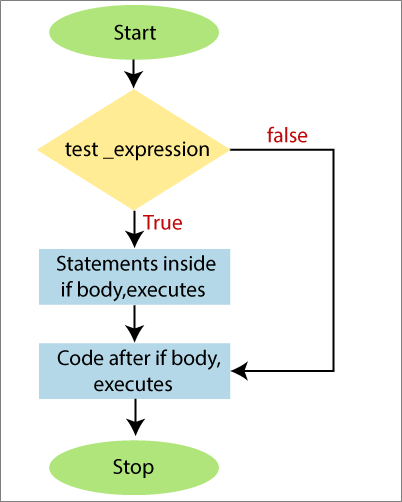# If Statement

When we need to execute the block of statements only when the specified condition is true, use an If statement.

This statement consists of a Boolean or logical expression followed by one or more statements. If the condition, which is a Boolean expression evaluates to True, then the statements inside the If statement will be executed. And if the condition evaluates to False, then the first statement after the end of if statement will be executed.

Statements inside the ‘if‘ body can be a single statement or a code of block enclosed by the curly brackets ‘{}‘.

## Syntax of If statement

The following is the syntax of If statement:

## Flow chart of If statement## Examples

The following examples illustrate the use of the If statement in PowerShell:

Example1: In this example, we will check the number in the variable ‘a‘ is even number. If a number is even, then print a message.

Output:

`The number is even  `

Example2: In this example, we will check the value of variable ‘a‘ is greater than the value of variable ‘b‘.

Output:

`The value of variable a is greater than the value of variable b  `

Example3: in this example, we will check the strings in variable ‘a‘ and ‘b‘ are same.

Output:

`True  `

Example4: In this example, we will display the square of the number. If the number is smaller than 10 and greater than zero.

Output:

`The square of \$p is \$z  `

Next TopicIf-Else Statement

Previous articleLearn MS Word Tutorial
Next articleWhat is PowerShell cmdlet